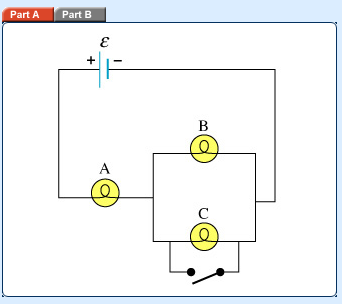# Problem: In this problem denotes the emf provided by the source, and is the resistance of each bulb.Bulbs A, B, and C in the figure are identical and the switch is an ideal conductor. How does closing the switch in the figure affect the potential difference?Check all that apply.a) The potential difference across A is unchanged.b) The potential difference across B drops to zero.c) The potential difference across A increases by 50%.d) The potential difference across B drops by 50%.

###### FREE Expert Solution

Closing the switch will direct the current to the path that has the least resistance comparison to B and C.

The entire current will flow through the switch and there will be no current flowing through B and C.###### Problem Details

In this problem denotes the emf provided by the source, and is the resistance of each bulb.

Bulbs A, B, and C in the figure are identical and the switch is an ideal conductor. How does closing the switch in the figure affect the potential difference?Check all that apply.

a) The potential difference across A is unchanged.

b) The potential difference across B drops to zero.

c) The potential difference across A increases by 50%.

d) The potential difference across B drops by 50%.